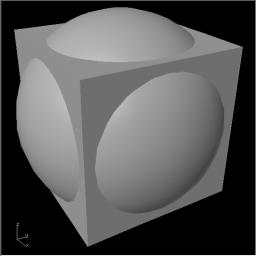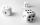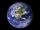# Cube from sphere

What largest surface area (in cm2) can have a cube that was cut out of a sphere with radius 43 cm?

Correct result:

A =  14792 cm2

#### Solution:We would be pleased if you find an error in the word problem, spelling mistakes, or inaccuracies and send it to us. Thank you!

Showing 1 comment:Math student
Nice puzzle. It took me a moment to realise my mistake and use 3D Pythagoras so I guess a hint might be helpful to others.Tips to related online calculators
Pythagorean theorem is the base for the right triangle calculator.

#### You need to know the following knowledge to solve this word math problem:

We encourage you to watch this tutorial video on this math problem:

## Next similar math problems:

• Percentage increaseIncrease number 400 by 3.5%
• Functions f,gFind g(1) if g(x) = 3x - x2 Find f(5) if f(x) = x + 1/2
• One halfOne half of ? is: ?
• Nineteenth memberFind the nineteenth member of the arithmetic sequence: a1=33 d=5 find a19
• PowersExpress the expression ? as the n-th power of the base 10.
• TransformerTransformer - U1 = 230 V, N1 = 300, N2 = 1,200, I1 = 4 A. Calculate the transformation ratio, voltage and current in the secondary coil.
• Factory and divisionsThe factory consists of three auxiliary divisions total 2,406 employees. The second division has 76 employees less than 1st division and 3rd division has 212 employees more than the 2nd. How many employees has each division?
• Volleyball8 girls wants to play volleyball against boys. On the field at one time can be six players per team. How many initial teams of this girls may trainer to choose?
• Two equationsSolve equations (use adding and subtracting of linear equations): -4x+11y=5 6x-11y=-5
• First manWhat is the likelihood of a random event where are five men and seven women first will leave the man?
• TreesA certain species of tree grows an average of 0.5 cm per week. Write an equation for the sequence that represents the weekly height of this tree in centimeters if the measurements begin when the tree is 200 centimeters tall.
• Cube surface areaThe surface of the cube was originally 216 centimeters square. The surface of the cube has shrunk from 216 to 54 centimeters sq. Calculate how much percent the edge of the cube has decreased.
• Three diceWhen you throw three dice was the sum total of the dice 10. The yellow dice fell one eye more than on the red and brown fell 3 eyes less than red. How many eyes fell on every dice?
• Cube cornersFrom cube of edge 5 cm cut off all vertices so that each cutting plane intersects the edges 1 cm from the nearest vertice. How many edges will have this body?
• Earth's surfaceThe greater part of the earth's surface (r = 6371 km) is covered by oceans; their area is approximately 71% of the Earth's surface. What is the approximate area of the land?
• Fit ballWhat is the size of the surface of Gymball (FIT - ball) with a diameter of 65 cm?
• Sphere vs cubeHow many % of the surface of a sphere of radius 12 cm is the surface of a cube inscribed in this sphere?